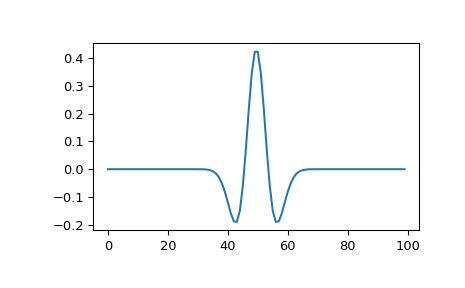# scipy.signal.ricker¶

scipy.signal.ricker(points, a)[source]

Return a Ricker wavelet, also known as the “Mexican hat wavelet”.

It models the function:

A (1 - x^2/a^2) exp(-x^2/2 a^2),

where A = 2/sqrt(3a)pi^1/4.

Parameters
pointsint

Number of points in vector. Will be centered around 0.

ascalar

Width parameter of the wavelet.

Returns
vector(N,) ndarray

Array of length points in shape of ricker curve.

Examples

>>> from scipy import signal
>>> import matplotlib.pyplot as plt

>>> points = 100
>>> a = 4.0
>>> vec2 = signal.ricker(points, a)
>>> print(len(vec2))
100
>>> plt.plot(vec2)
>>> plt.show()scipy.signal.qmf

scipy.signal.cwt